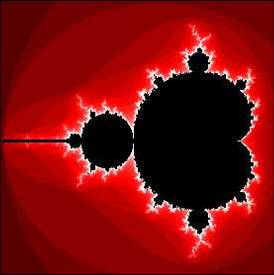Jules Henri Poincaré 1854-1912

# One of the most prominent figures in the history of mathematical physics is Henri Poincaré. He was born on April 29,1854 in Nancy and died on July 17, 1912 in Paris. He was a true genius! His contributions to physics and mathematics were vast. He was one of the early theoreticians who laid the groundwork for the area of relativity.Jules Henri Poincaré was one of the last universal mathematicians. He made contributions to the theory of complex functions, to number theory, algebraic and differential geometry, and to many branches of applied mathematics, including celestial mechanics.
He invented the notion of an abstract dynamical system in order to attack the question of the stability of the solar system, and, in the course of this study, invented the field of topology.
He believed that all physical laws should be "invariant under the Lorentz group." This insight, which expresses the hidden symmetries of Maxwell's equations, leads logically to Einstein's theory of special relativity when applied to Newtonian mechanics.
Henri Poincaré can be said to have been the originator of algebraic topology and of the theory of analytic functions of several complex variables.
Poincaré entered the Ecole Polytechnique in 1873 and continued his studies, as a student of Charles Hermite,at the Ecole des Mines, from which he received his doctorate in mathematics in 1879.
He was appointed to a chair of mathematical physics at the Sorbonne in 1881, a position he held until his death. cartier replica sale Before the age of 30 he developed the concept of automorphic functions which he used to solve second order linear differential equations with algebraic coefficients.
His Analysis situs, published in 1895, is an early systematic treatment of topology. Poincaré can be said to have been the originator of algebraic topology and of the theory of analytic functions of several complex variables.
He also worked in algebraic geometry and made a major contribution to number theory with work on Diophantine equations. In applied mathematics he studied optics, electricity, telegraphy, capillarity, elasticity, thermodynamics, potential theory, quantum theory, theory of relativity and cosmology. He is often described as the last universalist in mathematics.

In the field of celestial mechanics he studied the three-body-problem, and theories of light and electromagnetic waves. He is acknowledged as a co-discoverer, with Albert Einstein and Hendrik Lorentz, of the special theory of relativity.
His major works include Les Methods nouvelle de la méchanique celeste in three volumes published between 1892 and 1899 and Lecons de mecanique celeste (1905). In the first of these he aimed to completely characterise all motions of mechanical systems.
He invoked an analogy with fluid flow. He also showed that previous series expansions used in studying the 3-body problem were convergent, but not in general uniformly convergent, so putting in doubt the stability proofs of Lagrange and Laplace.
He also wrote many popular scientific articles including Science and Hypothesis (1901), Science and Method (1908), and The Value of Science (1904). hublot replica A quote from Poincaré is particularly relevant to this collection on the history of mathematics. In 1908 he wrote

The true method of foreseeing the future of mathematics is to study its history and its actual state.

The Poincaré conjecture is one of the most baffling and challenging unsolved problems in algebraic topology. Homotopy theory reduces topological questions to algebra by associating with topological spaces various groups which are algebraic invariants.

Poincaré introduced the fundamental group to distinguish different categories of two-dimensional surfaces. He was able to show that any 2-dimensional surface having the same fundamental group as the two-dimensional surface of a sphere is topologically equivalent to a sphere. cheap replica watches He conjectured that the result held for 3-dimensional manifolds and this was later extended to higher dimensions.

Surprisingly proofs are known for the equivalent of Poincaré's conjecture for all dimensions strictly greater than 3. No complete classification scheme for 3-manifolds is known, so, there is no list of possible manifolds that can be checked to verify that they all have different homotopy groups.
Poincaré was first to consider the possibility of chaos in a deterministic system, in his work on planetary orbits."A very small cause which escapes our notice determines a considerable effect that we cannot fail to see, and then we say that the effect is due to chance. rolex replica uk If we knew exactly the laws of nature and the situation of the universe at the initial moment, we could predict exactly the situation of the same universe at a succeeding moment. But even if it were the case that the natural laws had nolonger any secret for us, we could still know the situation approximately. If that enabled us to predict the succeeding situation with the same approximation, that is all we require, and we should say that the phenomenon had been predicted, that it is governed by the laws. rolex replica But is not always so; it may happen that small differences in the initial conditions produce very great ones in the finalphenomena. A small error in the former will produce an enormous error in the latter. Prediction becomes impossible...". (Poincaré)

Little interest was shown in his work until the modern study of chaotic dynamics began in 1963.Thus Poincaré can truly be said to be is the Father of the Theory of Chaos.